圖靈測試(Turing test)

在此測試的內容如下：圖靈機

其基本思法是用機器來模擬人們用紙筆進行數學運算的過程，圖靈將這樣的過程看作下列兩種簡單的動作：
1. 在紙上寫上或擦除某個符號
2. 把注意力從紙的一個位置移動到另一個位置

1. 一條無限長的紙帶TAPE。紙帶被劃分為一個接一個的小格子，每個格子上包含一個來自有限字母表的符號，字母表中有一個特殊的符號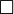$\square$表示空白。紙帶上的格子從左到右依次被編號為0, 1, 2, ...，紙帶的右端可以無限伸展。
3. 一套控制規則TABLE。它根據當前機器所處的狀態以及當前讀寫頭所指的格子上的符號來確定讀寫頭下一步的動作，並改變狀態暫存器的值，令機器進入一個新的狀態。
4. 一個狀態暫存器。它用來儲存圖靈機當前所處的狀態。圖靈機的所有可能狀態的數目是有限的，並且有一個特殊的狀態，稱為停機狀態。

其正式的定義則如下：

1.$Q$是狀態集合；
2.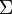$\Sigma$是輸入字母表，其中不包含特殊的空白符$\square$
3.$b \in \Gamma$空白符
4.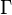$\Gamma$是帶字母表，其中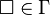$\square \in \Gamma$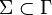$\Sigma \subset \Gamma$
5.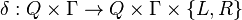$\delta : Q \times \Gamma \to Q \times \Gamma \times \{L, R\}$是轉移函式，其中$L, R$表示讀寫頭是向左移還是向右移；
6.$q_0 \in Q$是起始狀態；
7.$q_{accept} \in Q$是接受狀態。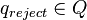$q_{reject}\in Q$是拒絕狀態，且$q_{reject}\neq q_{accept}$

電影「模仿遊戲」

(同期間還有荷蘭的亞歷山大 寇赫(Alexander Koch)以及瑞典的亞維 當姆(Arvid Damm)與美國的發明家愛德華 赫本(Edward Hebern)，但三位皆因沒有遇到賞識的金主而作罷。)

//所以沒有Christopher唷~~//